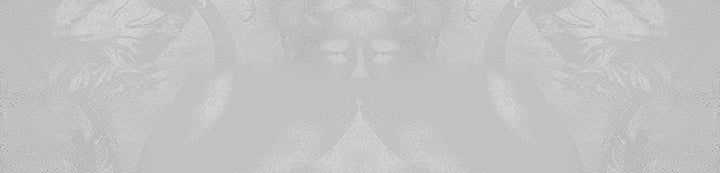1 - 1 of 1 Posts

hyphy03

·
Registered
Joined
·
26,682 Posts
mordeaci said:
Waka Flocka fans that have only heard Hard in Da Paint and No Hands <<<<<<<<<<<<<<<<<

Based God fans that only know his swag songs <<<<<<<<<<<<<<<<<<<<<<<

Pusha T fans who don't know about his Clipse work <<<<<<<<<<<<<<<<<<<<<<

Rick Ross fans who generalize him on his old work with DJ Khaled <<<<<<<<<<<<<<<<<<<

Fans of 'Ye and Jeezy that only know their top singles <<<<<<<<<<<<<<<<<<<<<<<<<<<<<
Weezy_KB24 said:
Fans of Weezy that only know Lollipop, A Milli, Got Money, HTL, and 6F7F <<<<<<<<<<<

1 - 1 of 1 Posts